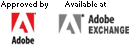Why our extensions?
- High Quality, Tested and Approved
- Unlimited Domain License
- Dedicated Support Team
- Easy to Install and Use# Calculated Fields

## Dreamweaver Extension for MX, DW8, CS3, CS4, CS5, CS6, CC, ...

Calculated Field is an extension for Adobe Dreamweaver. It allows to easily insert dynamically calculated fields in your form; you can select different fields that will be involved in an equation of the calculated field and you will be able to select the INPUT fields of TEXT or HIDDEN type which will store the result of the introduced equation. With Calculated Field, every time that one of the fields that is involved in the equation changes its value, the result will be reflected automatically in the field that stores the equation result.

Note: If you are looking for a calculated fields plugin for WordPress, please check our Calculated Fields for WordPress.

When we create forms, we often need to have a field that depends on what was selected previously in other fields; for example, in purchase forms, price depends on the number of items; in shipping forms, it may depend on the zip code of the destination; in rental forms, on the contract length. In all these cases, we need a dynamically calculated field, whose value is updated automatically, whenever the value of another field in the form is changed.

Requirements

The requirements are simple: Adobe Dreamweaver MX or later (including MX 2004, DW 8, DW CS3, DW CS4, DW CS5, DW CS6, DW CC,...).

Samples & Demo

Calculate Average
Please introduce number value for class. The value of average field is ( Class A + Class B + Class C + Class D ) / 4

Class A

Class B

Class C

Class D

Average
Help & FAQ
Installation

Calculated Field is distributed as an Adobe Dreamweaver MX (or later) extension, packed in a file named HDW_CalcField.mxp.

To install it, you only need to execute that file or use Adobe Extension Manager.

Once installed, the Calculated Field can be accessed, after restarting Dreamweaver, via the Menu Option "INSERT > Form > Calculated Field", or through "InsertBar > Forms Tag > Calculated Field".

Notes

To use the Calculated Field extension on a webpage being developed with Adobe Dreamweaver MX (or later), you will need to:

• Save the webpage where a Calculated Field will be used.
• Select the form where you wish to insert a Calculated Field.

By executing Calculated Field extension (See how to access Calculated Field under Installation), a setup window will open up and you will be able to set the equation.

The fields of the setup window (Fig 1), are:ADD ELEMENT TO EQUATION: List of ID's of the form fields that can be selected to form part of the equation. The fields that can form part of the equation are: INPUT fields with the exception of BUTTON, RESET, SUBMIT, IMAGE and FILE types; SELECT fields with the exception of the fields that allow multiselection.

SELECT THE FIELD THAT WILL STORE THE EQUATION RESULT: List of the ID's of the INPUT fields of HIDDEN or TEXT type, that are part of the form. The selected field in this list will contain the result of the equation and will be updated dynamically as soon as the values of the fields participating in the equation change. The field that is selected to receive the result of the equation cannot form part of the equation itself.

RESULTING EQUATION: Equation that will be employed to obtain the value of the dynamically calculated field. The resulting equation only can be constituted by the fields that are part of the form and that are represented by numeric values. The equation will only admit arithmetic operators +, -, *, /, mathematical functions and grouping operators (,). Here's an example of a resulting equation: (Field1+Field2)/2 + Field3, where Field1, Field2, and Field3 are fields that are part of the form where a calculated field has been inserted.

Mathematical functions:

abs(number). Returns the absolute value of the number passed as a parameter.

ceil(number). Returns the next higher integer that is greater than or equal to the number passed as a parameter.

floor(number). Returns the next lower integer that is less than or equal to the number passed as a parameter.

round(number). Returns an integer that follows rounding rules. If the value of the passed parameter is greater than or equal to x.5, the returned value is x+1; otherwise the returned value is x.

prec(number1, number2). Returns the value of the number passed in the first parameter with so many decimal digits as the number passed in the second parameter.

log(number). Returns the natural logarithm (base e) of the number passed as a paramenter.

pow(number1, number2). Returns the value of the first parameter raised to the power of the second parameter.

sqrt(number). Returns the square root of the number passed as a paramenter.

max(number1, number2). Returns the greater value of the two parameters.

min(number1, number2). Returns the lesser value of the two parameters.

The extension has an area in the lower part that provides help hints; the hints appear simultaneously during the setup process.

When the calculated field is inserted, a function named CalcField _form is placed to follow the form. It contains all the equations of the calculated fields that have been introduced into the form identified by <form id> and a new folder named Jscript, which will contain a javascript file CalculateField.js

The directory JScript, along with the file that it contains, must be published together with your website at the same location relative to the webpage that contains a Calculated Field.